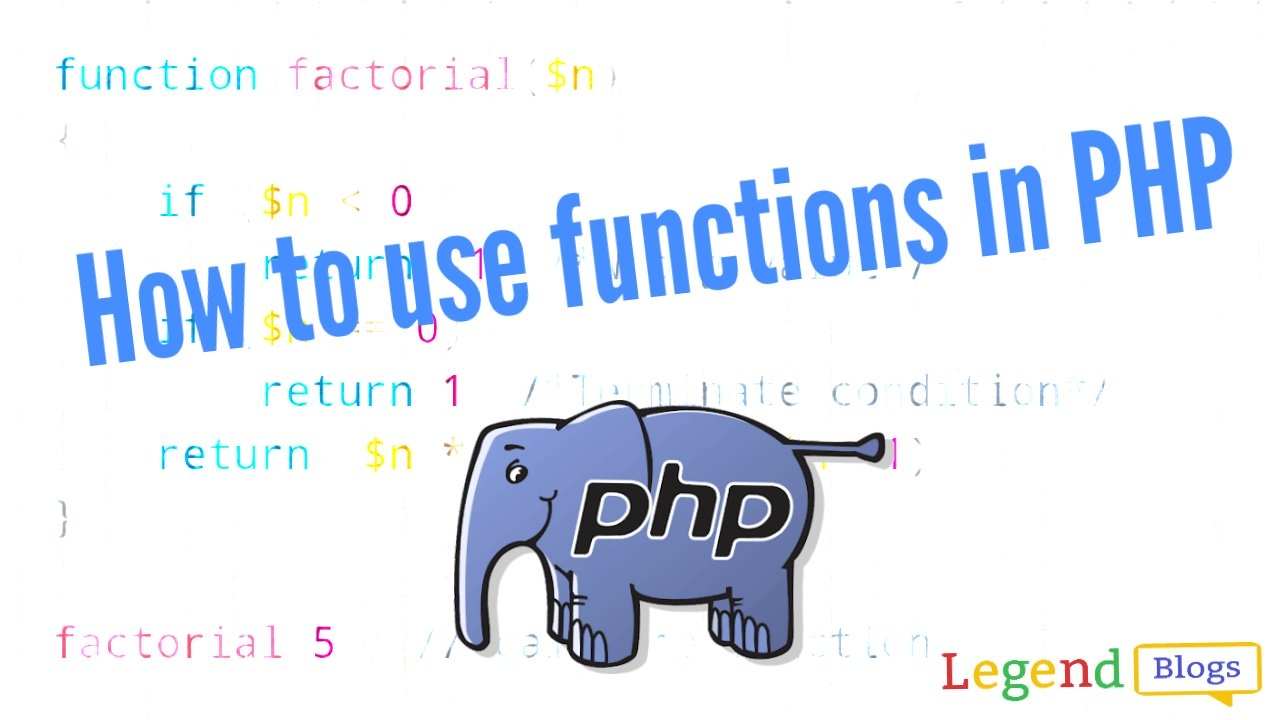# Functions in PHP## PHP Functions:

PHP function is a piece of code that can be reused many times. PHP functions are similar to other programming languages. More than thousands pr-define functions are available in PHP. There are many advantage of PHP Functions like Code Reusability, Easy to understand, Less Code etc. A user-defined function declaration starts with the word Function. A function name can start with a letter or underscore (not a number). The best practise is given, the function a name that reflects what the function does.

``````function <function_name>(){

....

}``````

In PHP, we can define Simple function, Parameterized function and Recursive function also.

Let's take a exmpale for all:

## Simple function:

In the example, we create a function named "myFunction()". The opening curly brace  { indicates the beginning of the function code and the closing curly brace } indicates the end of the function. Function have some data for print:

``````function myFunction() {
echo "Hello world!";
}

myFunction(); // call the function``````

Output of the function:

Hello world!

## Parameterized function:

In the example, You can pass any number of parameters inside a function. These passed parameters act as variables inside your function.. Function have some data for print:

``````function add(\$x, \$y) {
\$sum = \$x + \$y;
echo "Sum of two numbers is = \$sum <br><br>";
}

Output of the function:

Sum of two numbers is = 32

## Recursive function:

PHP also supports recursive function call like C/C++. In such case, we call current function within function. It is also known as recursion. Let's take a exmpale for factorial of a number :

``````function factorial(\$n)
{
if (\$n < 0)
return -1; /*Wrong value*/
if (\$n == 0)
return 1; /*Terminate condition*/
return (\$n * factorial (\$n -1));
}

factorial(5); // call the function``````

Output of the function:

120

## Call By Reference function:

In call by reference, actual value is modified if it is modified inside the function. In such case, you need to use & (ampersand) symbol with formal arguments. In this example, variable \$str is passed to the adder function where it is concatenated with 'Call By Reference' string. Here, printing \$str variable results 'This is Call By Reference'.

``````function reference(&\$str2)
{
\$str2 .= 'Call By Reference';
}
\$str = 'This is ';
reference(\$str);  // call the function
echo \$str;   ``````

Output of the function:

`This is Call By Reference`

• 26 May 2023

• 24 May 2023

• 20 Mar 2023